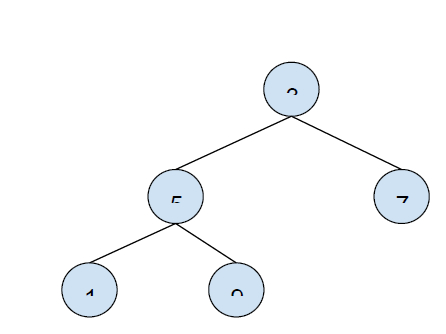# Write a Program to Find the Maximum Depth or Height of a Tree in C++

In this problem, we are given a binary tree. Our task is to write a program to find the maximum depth or height of the given tree.

Let’s take an example to understand the problem,The height of the tree is 3.

To find the maximum height of a tree, we will check the heights of its left and right subtree and add one to the maximum of both. This is a recursive process that will continue this the last node is of the tree is found and one is added progressively to find the height of the sub-trees.

## Above example solved using this method.

Finding the height of tree i.e. height(3) = max(height(5),height(7)) + 1.

For this, we will calculate the height of nodes with values 5 and 7.

height(5) = max(height(1),height(9)) + 1 and

height(7) = 1, it has no subtree that can be formed.

Similarly, height(1) = height(9) = 1

height(5) = max(1,1) +1 = 2

height(3) = max(height(5),height(7)) + 1 = max(2,1) + 1 = 3.

So the height becomes 3.

Program to illustrate the solution,

## Example

Live Demo

#include <iostream>
using namespace std;
class node {
public:
int data;
node* left;
node* right;
};
int height(node* node) {
if (node == NULL)
return 0;
else {
int lDepth = height(node->left);
int rDepth = height(node->right);
if (lDepth > rDepth)
return(lDepth + 1);
else return(rDepth + 1);
}
}
node* insertNode(int data) {
node* Node = new node();
Node->data = data;
Node->left = NULL;
Node->right = NULL;
return(Node);
}
int main() {
node *root = insertNode(4);
root->left = insertNode(5);
root->right = insertNode(0);
root->left->left = insertNode(1);
root->left->right = insertNode(9);
cout<<"The height of the given binary tree is "<<height(root);
return 0;
}

## Output

The height of the given binary tree is 3